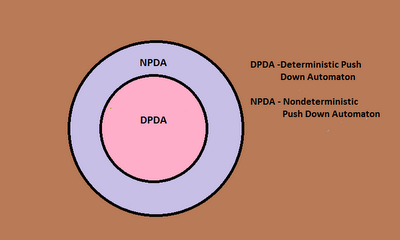## Friday, 30 November 2012

### Different Types of Automata in Language Theory

It is very important for every one interested in Computer Science to understand various Automata in language theory. Often there is a big confusion about the relationship between various Automaton. There are different Automata, but the most important ones with practical applications are the following. Turing Machine (TM), Linear Bounded Automata (LBA), Push Down Automata (PDA) and Finite Automata (FA).

Each one of theses Automata are having a Deterministic and non deterministic variant associated with them. It is often confusing since there are eight different types of Automata to account for. Here I have included simple Venn diagrams to show the hierarchy of Automata. The outer most set is the one with the most power. Here power is the ability to accept strings belonging to complicated languages. The Automata with more power can accept more complicated strings. Remember here I have used layman's terms.

The Venn diagram given below shows a power comparison between the four types of Automata.A hierarchy of different Automata

Now let us see how a Deterministic Turing Machine (DTM) is related to  a Non deterministic Turing Machine (NTM). The Venn diagram given below gives an idea about the two Automata.Turing Machine Hierarchy

Next is the least known of all the four Automata. The Linear Bounded Automata (LBA). Well here we don't have a clear answer about the relationship between a Deterministic LBA (DLBA) and a Non deterministic LBA (NLBA). It is still an unsolved problem in Computer Science. So I give a Venn diagram showing both the possibilities.Here is an Open Problem. You could give it a try.
Now we have Push Down Automata (PDA). The relationship between Deterministic PDA (DPDA) and Non deterministic PDA (NPDA) is shown in the Venn diagram given below.DPDA and NPDA are having different powers

Finally we have Finite Automata. Both Non deterministic Finite Automata (NFA) and Deterministic Finite Automata (DFA) are having equal powers. The Venn diagram below shows their relationship.NFA and DFA

The last figure given below shows the relationship between various Grammars associated with the above mentioned Automaton.Hierarchy of Grammars